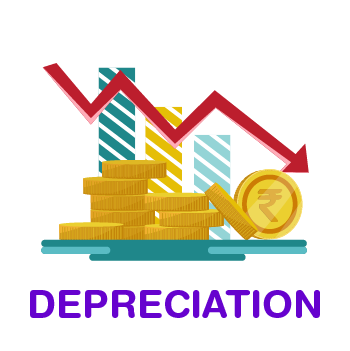# What is Depreciation? Straight Line Method

## Meaning of Depreciation

Depreciation can be defined as a continuing, permanent and gradual decrease in the book value of fixed assets. This type of shrinkage is based on the cost of assets utilised in a firm and not on its market value.## Features of Depreciation

Following are the 3 principal features of depreciation:

• Depreciation is a decrease in the book value of fixed assets.
• Depreciation involves loss of value of assets due to the passage of time and obsolescence.
• Depreciation is an ongoing process until the end of the life of assets.

## Causes of Depreciation

1. Wear and Tear due to Use or Passage of Time: Wear and tear is nothing but deterioration and the following decrease in the value of an asset, resulting from its use in business operations for earning revenue.
2. Expiration of Legal Rights: Some categories of assets lose their value after the agreement directing their use in business comes to an end after the expiry of the predetermined period.
3. Obsolescence: Obsolescence is another factor driving to the depreciation of fixed assets. In common language, obsolescence means being “out-of-date”. Obsolescence refers to an actual asset becoming outdated on account of the availability of a better type of asset.
4. Abnormal Factors: Drop in the use of the asset may be caused by abnormal factors. Namely, accidents due to the earthquake, fire, floods, etc., Accidental loss is permanent but not continuing.

### Depreciation Formula:

1. Annual amount of depreciation under Straight Line Method

Annual Depreciation      =

$$\begin{array}{l}\frac{Original\, Cost\, -\, Estimated\, Scrap\, Value}{Estimated\, Useful\, Life}\end{array}$$

2. Rate of depreciation on original cost

Rate of depreciation =

$$\begin{array}{l}\frac{Annual\, Depreciation}{Original\, Cost\, of\, Asset}\, X\, 100\end{array}$$

 Advantages Disadvantages It is a very simple method of calculating depreciation. Under this method, Asset can be depreciated up to the net scrap value or zero value. Under this method, the same amount is charged as depreciation in Profit & Loss Account. Under this method book value of the asset will be charged more for maintenance and repair in the final years as compared to initial years. It is difficult to ascertain a suitable rate of depreciation. It is not suitable for assets having long life and high value.

## Methods of Calculating Depreciation

Straight Line Method (SLM)

Under the depreciation Straight Line Method, a fixed depreciation amount is charged annually, during the lifetime of an asset. The amount of annual depreciation is computed on Original Cost and it remains fixed from year to year. This method is also known as the ‘Original Cost method’ or ‘Fixed Instalment method’.

Written Down Value Method (WDV)

Under the Written Down Value method, depreciation is charged on the book value (cost –depreciation) of the asset every year. Under the WDV method, book value keeps on reducing so, annual depreciation also keeps on decreasing. This method is also known as ‘Diminishing Balance Method’ or ‘Reducing Instalment Method’.

### Straight Line Method vs Written Down Value Method

 Straight Line Method Written Down Value Method Basis of Charging Depreciation Depreciation is calculated on the original cost of fixed assets. Depreciation is calculated on the book value of fixed assets. Amount of Annual Depreciation The amount of annual depreciation is fixed for all years of useful life. The amount of depreciation declines year after year. Recognition by Income Tax The Straight Line Method is not recognised by the Income Tax Department. The Written Down Value method is recognised by the Income Tax Department. Cost of Depreciation and Repairs The combined cost on account of depreciation and repairs is lower in the initial years and higher in the later years. The combined cost on account of depreciation and repairs remains, more or less, equal throughout the life of the asset.

### Theory Questions:

 Q.1- What are the other concepts similar to depreciation? Answer: Depletion The term ‘Depletion’ is used with regard to the ‘Natural Resources’ like oil wells, Mines etc. When natural resources are extracted and their stock value is reduced. This reduction is termed as depletion. Amortisation The term ‘Amortisation’ is used with regard to ‘Intangible Assets’. Amortisation refers to writing off the cost of intangible assets like patents, copyright, trademarks, franchises, etc. Obsolescence It refers to a decline in the value of assets due to innovation or improved techniques, changes in the taste or fashion of the existing asset.
 Q.2- State the objectives of depreciation. Answer: Objectives of Depreciation To ascertain the correct cost of production To retain funds for a replacement. To ascertain the correct Profit or Loss. To show a true and fair view of the financial position.
 Q.3- List the factors required for calculating the amount of depreciation. Also give the components of original cost. Answer: (a) Factors/elements required to calculate depreciation The original cost of the assets. Estimated residual value or scrap value. The estimated useful life of the assets. (b) Original cost Original cost = Purchase price + Freight + Installation cost + Any other expenses incurred before the asset is put to use for the first time. In the case of second-hand assets, even expenses incurred on repair & overhauling, before putting it to use, are also included in the original cost.

 Multiple Choice Questions Q.1- Amortisation refers to writing off the cost of: a. Fixed Assets b. Tangible Assets c. Intangible Assets d. All of the above Q.2- ________refers to decline in the value of assets due to Innovation or improved techniques, changes in the taste or fashion of the existing asset. a. Intangible Assets b. Obsolescence c. Amortisation d. Depreciation Q.3- Depreciation is a ________expense. a. Non-cash b. Cash c. Credit d. All of the above